# Lesson 1 Tidy Spatial Analysis

## 1.1 Overview

Questions

• How to elegantly conduct complex spatial analysis by chaining operations?
• What is the percent area of water by region across the United States?

Objectives

• Use the `%>%` operator (aka “then” or “pipe”) to pass output from one function into input of the next.
• Calculate metrics on spatial attributes.
• Aggregate spatial data with metrics.
• Display a map of results.

## 1.2 Prerequisites

R Skill Level: Intermediate - you’ve got basics of R down.

You will use the `sf` package for vector data along with the `dplyr` package for calculating and manipulating attribute data.

``````# load packages
library(tidyverse)  # load dplyr, tidyr, ggplot2 packages
library(sf)         # vector reading & analysis

# set working directory to data folder
# setwd("pathToDirHere")``````

## 1.3 States: read and plot

Similar to Lesson 9: Handling Spatial Projection & CRS in R, we’ll start by reading in a polygon shapefile using the `sf` package. Then use the default `plot()` function to see what it looks like.

``````# read in states

# plot the states
plot(states)``````
``````## Warning: plotting the first 9 out of 10 attributes; use max.plot = 10 to
## plot all``````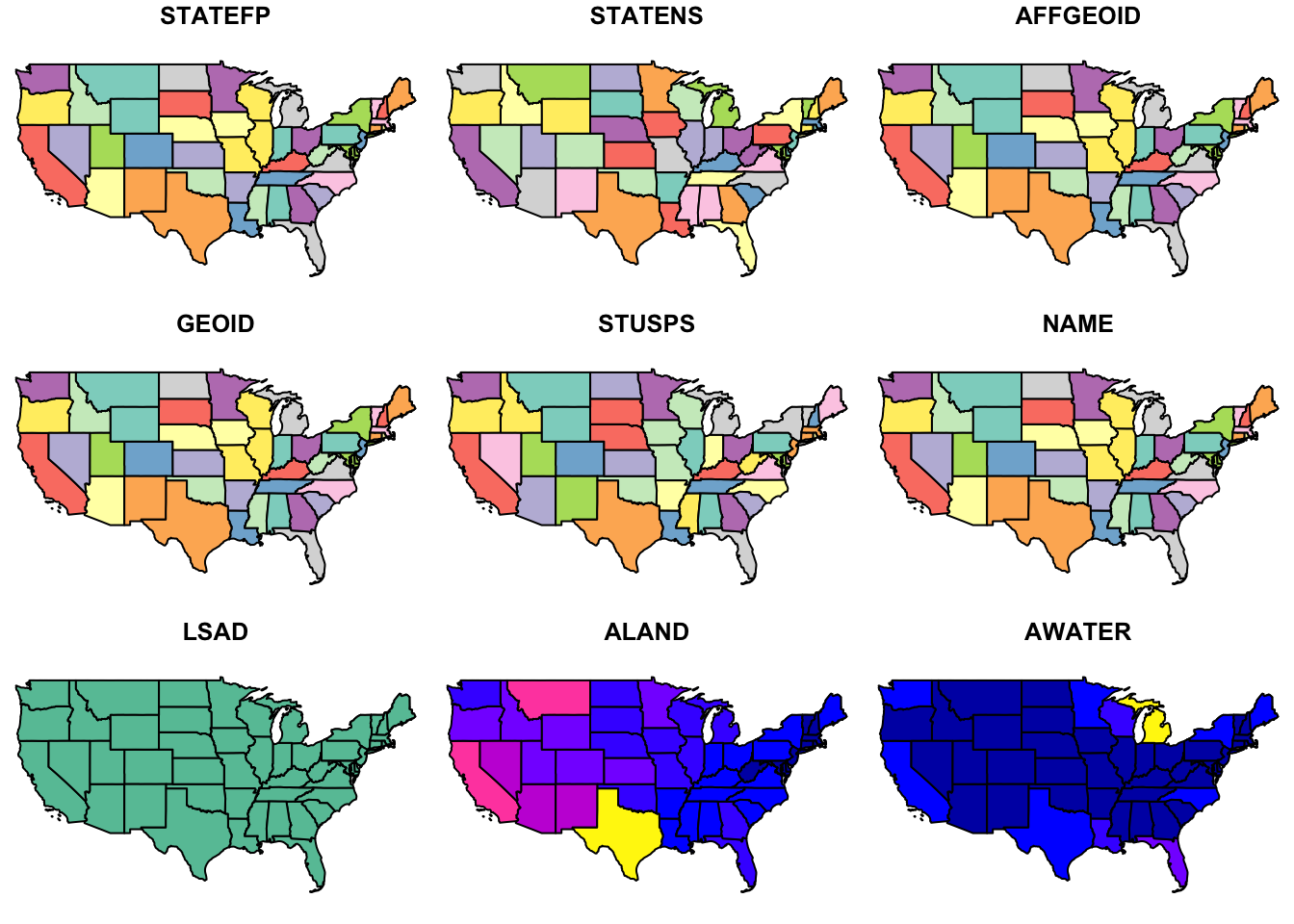Notice the default plot on `sf` objects outputs colorized values of the first 9 of 10 columns. Use the suggestion from the warning to plot the 10th column.

``````# plot 10th column
plot(states, max.plot = 10)``````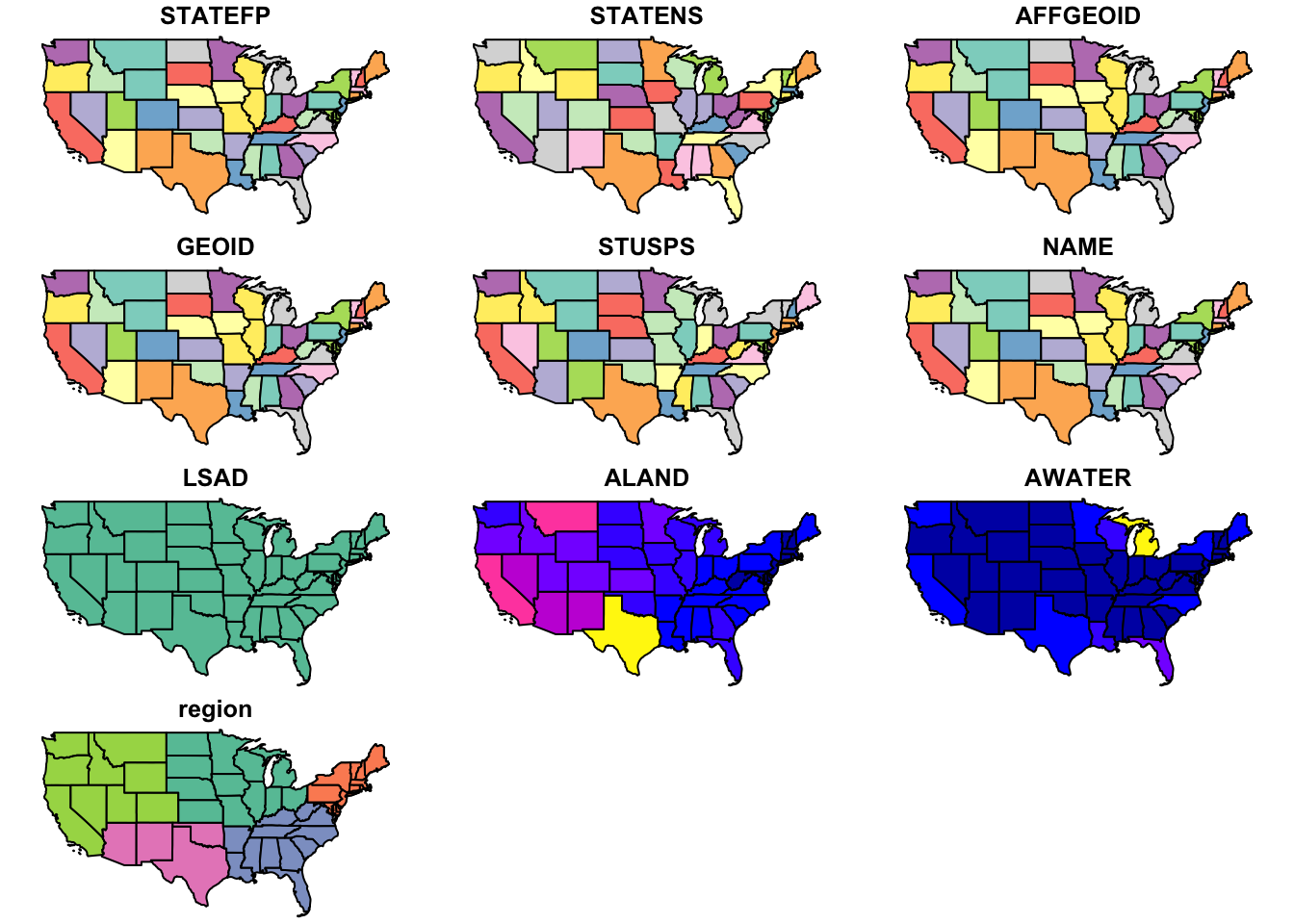``````# show columns of the data frame
names(states)``````
``````##   "STATEFP"  "STATENS"  "AFFGEOID" "GEOID"    "STUSPS"   "NAME"
##   "LSAD"     "ALAND"    "AWATER"   "region"   "geometry"``````
``````# look at table
glimpse(states)``````
``````## Observations: 58
## Variables: 11
## \$ STATEFP  <chr> "06", "11", "12", "13", "16", "17", "19", "21", "22",...
## \$ STATENS  <chr> "01779778", "01702382", "00294478", "01705317", "0177...
## \$ AFFGEOID <chr> "0400000US06", "0400000US11", "0400000US12", "0400000...
## \$ GEOID    <chr> "06", "11", "12", "13", "16", "17", "19", "21", "22",...
## \$ STUSPS   <chr> "CA", "DC", "FL", "GA", "ID", "IL", "IA", "KY", "LA",...
## \$ NAME     <chr> "California", "District of Columbia", "Florida", "Geo...
## \$ LSAD     <chr> "00", "00", "00", "00", "00", "00", "00", "00", "00",...
## \$ ALAND    <dbl> 403483823181, 158350578, 138903200855, 148963503399, ...
## \$ AWATER   <dbl> 20483271881, 18633500, 31407883551, 4947080103, 23977...
## \$ region   <chr> "West", "Northeast", "Southeast", "Southeast", "West"...
## \$ geometry <simple_feature> MULTIPOLYGONZ(((-118.593969..., MULTIPOLYG...``````
``````# convert to tibble for nicer printing
as_tibble(states)``````
``````## Simple feature collection with 58 features and 10 fields
## geometry type:  MULTIPOLYGON
## dimension:      XYZ
## bbox:           xmin: -124.7258 ymin: 24.49813 xmax: -66.9499 ymax: 49.38436
## epsg (SRID):    4326
## proj4string:    +proj=longlat +datum=WGS84 +no_defs
## # A tibble: 58 x 11
##    STATEFP  STATENS    AFFGEOID GEOID STUSPS                 NAME  LSAD
##      <chr>    <chr>       <chr> <chr>  <chr>                <chr> <chr>
##  1      06 01779778 0400000US06    06     CA           California    00
##  2      11 01702382 0400000US11    11     DC District of Columbia    00
##  3      12 00294478 0400000US12    12     FL              Florida    00
##  4      13 01705317 0400000US13    13     GA              Georgia    00
##  5      16 01779783 0400000US16    16     ID                Idaho    00
##  6      17 01779784 0400000US17    17     IL             Illinois    00
##  7      19 01779785 0400000US19    19     IA                 Iowa    00
##  8      21 01779786 0400000US21    21     KY             Kentucky    00
##  9      22 01629543 0400000US22    22     LA            Louisiana    00
## 10      24 01714934 0400000US24    24     MD             Maryland    00
## # ... with 48 more rows, and 4 more variables: ALAND <dbl>, AWATER <dbl>,
## #   region <chr>, geometry <simple_feature>``````
``names(states)``
``````##   "STATEFP"  "STATENS"  "AFFGEOID" "GEOID"    "STUSPS"   "NAME"
##   "LSAD"     "ALAND"    "AWATER"   "region"   "geometry"``````
``````# inspect the class(es) of the states object
class(states)``````
``##  "sf"         "tbl_df"     "tbl"        "data.frame"``

The class of the `states` object is both a simple feature (`sf`) as well as a data frame, which means the many useful functions available to a data frame (or “tibble”) can be applied.

To plot the column of interest, feed the “slice” of that column to the `plot()` function.

``plot(states['region'])``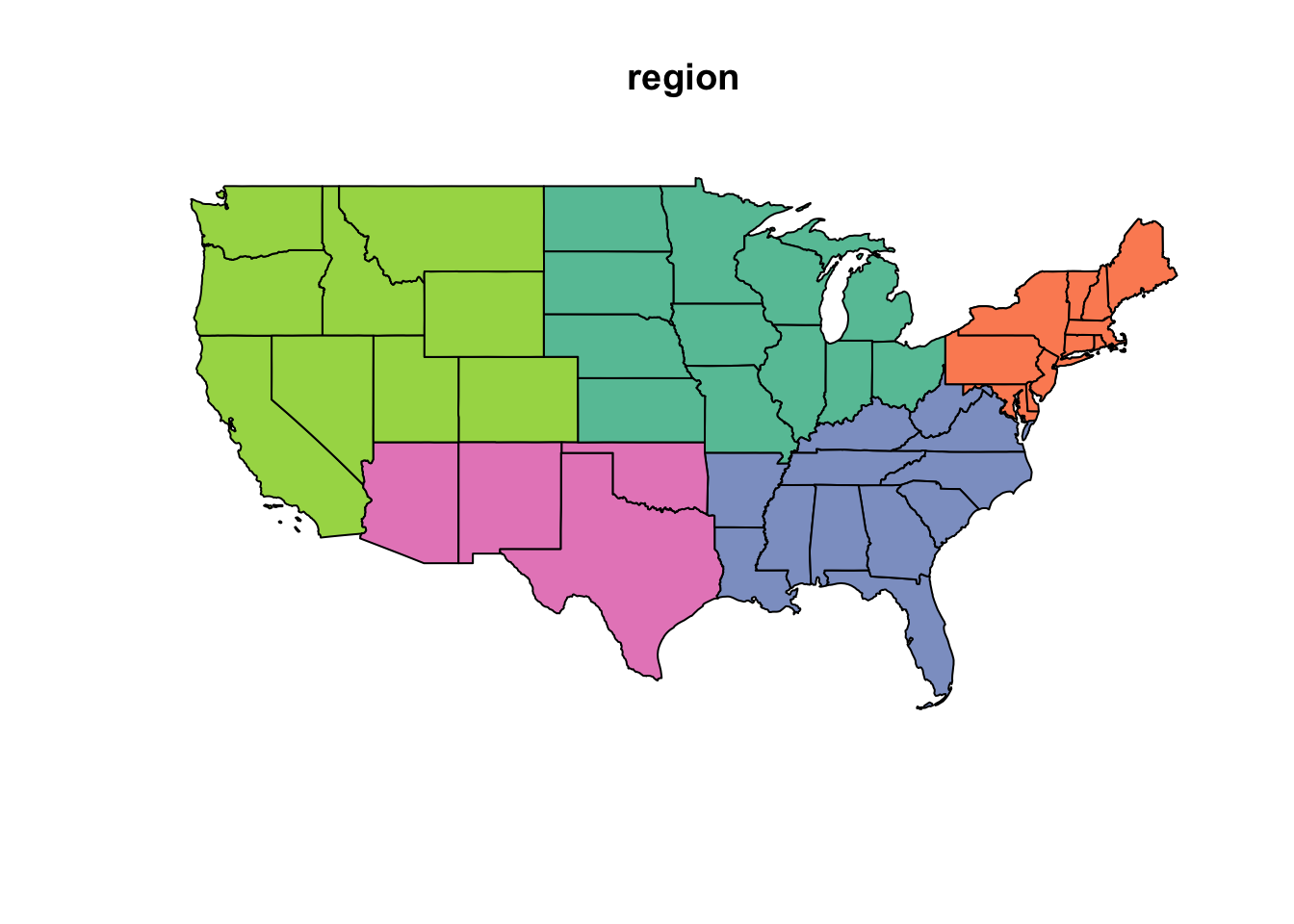Question: To motivate the spatial analysis for the rest of this lesson, you will answer this question: “What is the percent water by region?

## 1.4 Challenge: analytical steps?

Outline a sequence of analytical steps needed to arrive at the answer.

1. Sum the area of water (`AWATER`) and land (`ALAND`) per region.
2. Divide the area of water (`AWATER`) by the area of land (`ALAND`) per region to arrive at percent water.
3. Show table of regions sorted by percent water.
4. Show map of regions by percent water with a color ramp and legend.

## 1.5 Regions: calculate % water

• Use the `%>%` operator (aka “then” or “pipe”) to pass output from one function into input of the next.
• In RStudio, see menu Help > Keyboard Shortcuts Help for a shortcut to the “Insert Pipe Operator”.
• Calculate metrics on spatial attributes.
• Aggregate spatial data with metrics.
``````regions = states %>%
group_by(region) %>%
summarize(
water = sum(AWATER),
land  = sum(ALAND)) %>%
mutate(
pct_water = water / land * 100 %>% round(2))

# object
regions``````
``````## Simple feature collection with 5 features and 4 fields
## geometry type:  GEOMETRY
## dimension:      XYZ
## bbox:           xmin: -124.7258 ymin: 24.49813 xmax: -66.9499 ymax: 49.38436
## epsg (SRID):    4326
## proj4string:    +proj=longlat +datum=WGS84 +no_defs
## # A tibble: 5 x 5
##      region        water         land pct_water          geometry
##       <chr>        <dbl>        <dbl>     <dbl>  <simple_feature>
## 1   Midwest 184383393833 1.943869e+12  9.485380 <MULTIPOLYGON...>
## 2 Northeast 108922434345 8.690661e+11 12.533273 <MULTIPOLYGON...>
## 3 Southeast 103876652998 1.364632e+12  7.612063 <MULTIPOLYGON...>
## 4 Southwest  24217682268 1.462632e+12  1.655761 <POLYGONZ((-9...>
## 5      West  57568049509 2.432336e+12  2.366780 <MULTIPOLYGON...>``````

Notice the geometry in the column. To remove the geometry column pipe to `st_set_geometry(NULL)`. To arrange in descending order use `arrange(desc(pct_water))`.

``````# table
regions %>%
st_set_geometry(NULL) %>%
arrange(desc(pct_water))``````
``````## # A tibble: 5 x 4
##      region        water         land pct_water
##       <chr>        <dbl>        <dbl>     <dbl>
## 1 Northeast 108922434345 8.690661e+11 12.533273
## 2   Midwest 184383393833 1.943869e+12  9.485380
## 3 Southeast 103876652998 1.364632e+12  7.612063
## 4      West  57568049509 2.432336e+12  2.366780
## 5 Southwest  24217682268 1.462632e+12  1.655761``````

## 1.6 Regions: plot

Now plot the regions.

``````# plot, default
plot(regions['pct_water'])``````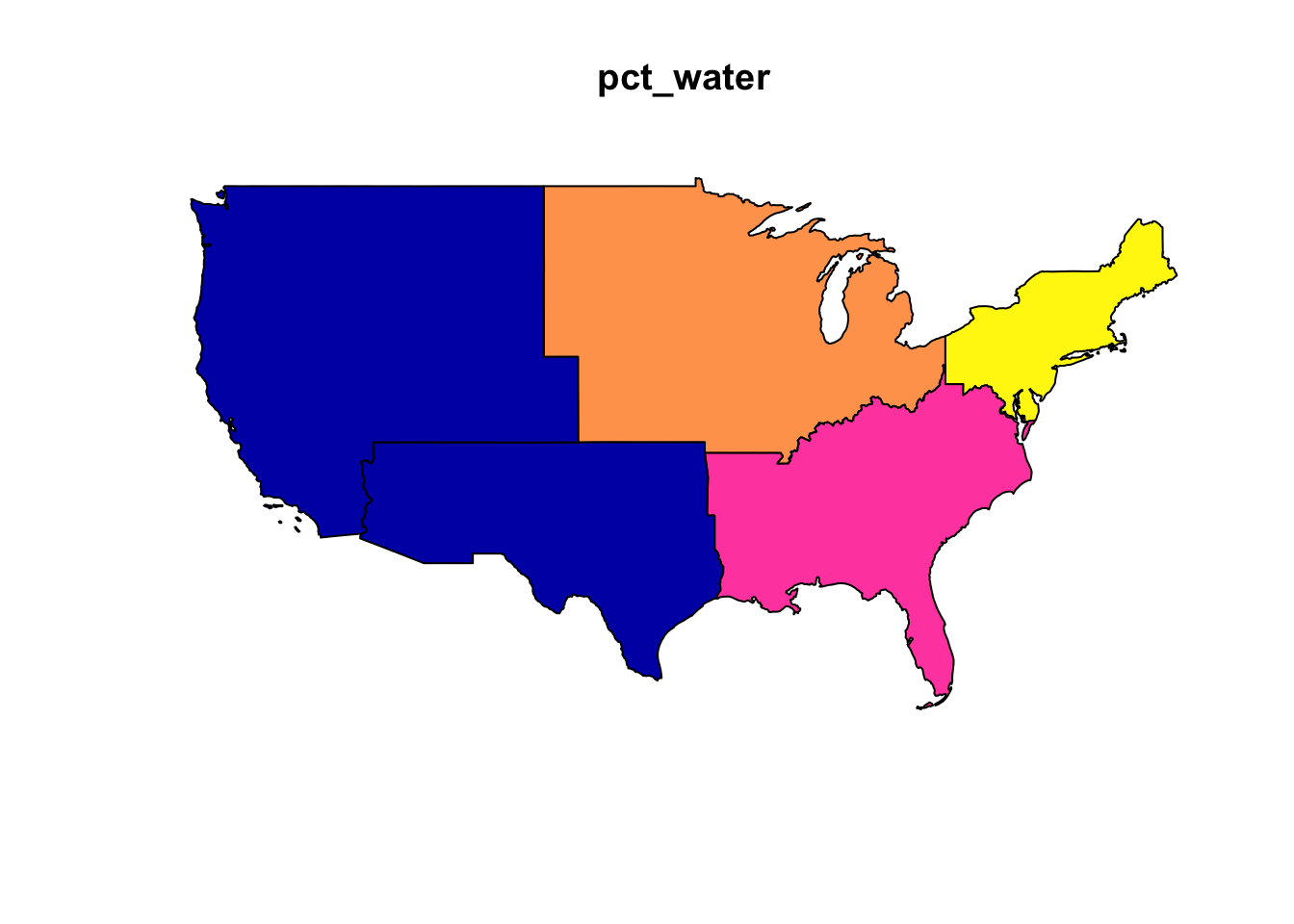## 1.7 Regions: ggplot

The `ggplot2` library can visualise sf objects.

``````# plot, ggplot
ggplot(regions) +
geom_sf(aes(fill = pct_water)) +
scale_fill_distiller(
"pct_water", palette = "Spectral", direction=1,
guide = guide_legend(title = "% water", reverse=T)) +
theme_bw() +
ggtitle("% Water by US Region")``````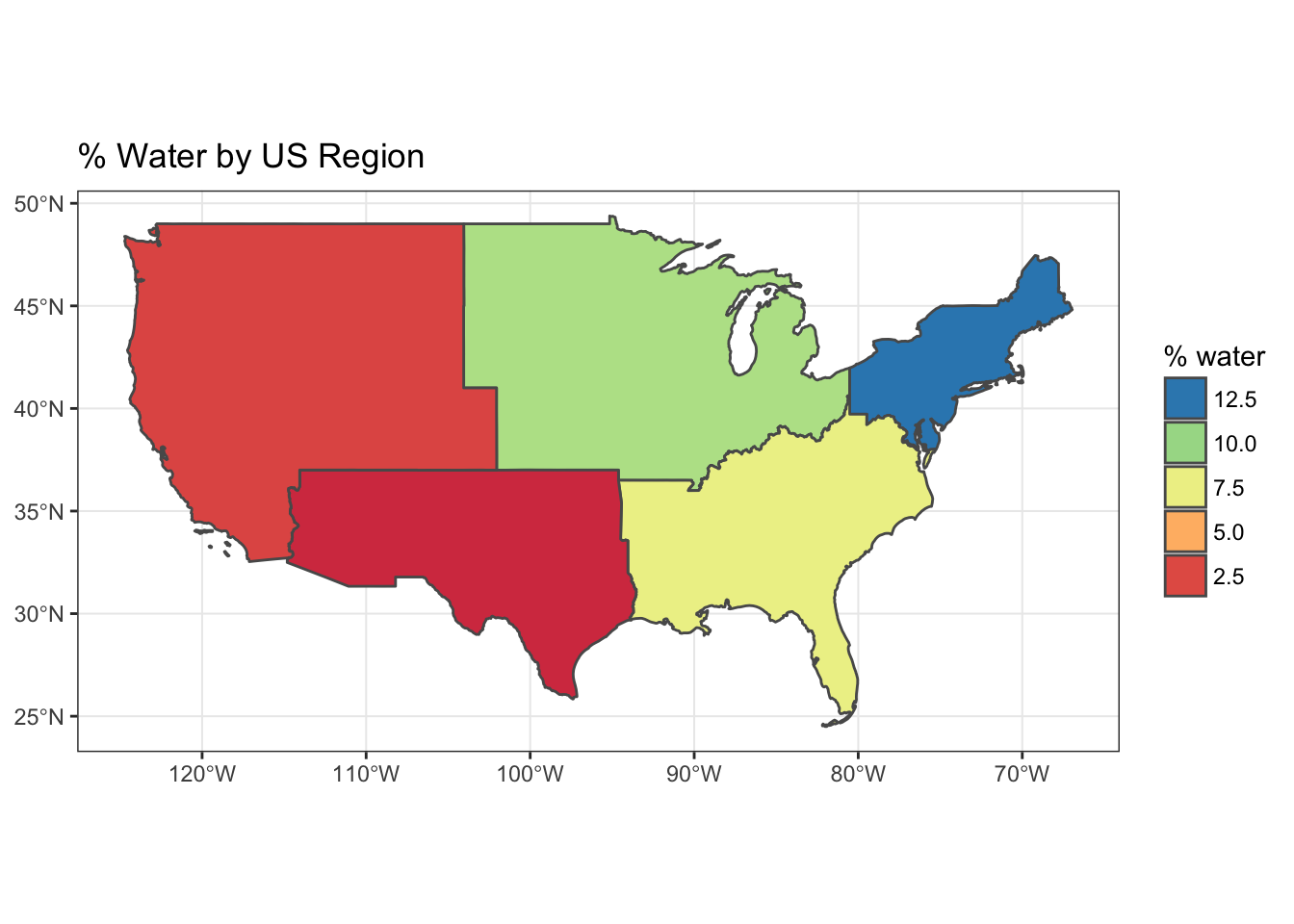## 1.8 Regions: recalculate area

So far you’ve used the `ALAND` column for area of the state. But what if you were not provided the area and needed to calculate it? Because the `states` are in geographic coordinates, you’ll need to either transform to an equal area projection and calculate area, or use geodesic calculations. Thankfully, the `sf` library provides area calculations with the `st_area()` and uses the `geosphere::distGeo()` to perform geodesic calculations (ie trigonometric calculation accounting for the spheroid nature of the earth). Since the `states` data has the unusual aspect of a z dimension, you’ll need to first remove that with the `st_zm()` function.

``````library(geosphere)
library(units)

regions = states %>%
mutate(
water_m2 = AWATER %>% set_units(m^2),
land_m2  = geometry %>% st_zm() %>% st_area()) %>%
group_by(region) %>%
summarize(
water_m2 = sum(water_m2),
land_m2  = sum(land_m2)) %>%
mutate(
pct_water = water_m2 / land_m2)

# table
regions %>%
st_set_geometry(NULL) %>%
arrange(desc(pct_water))``````
``````## # A tibble: 5 x 4
##      region         water_m2          land_m2    pct_water
##       <chr>          <units>          <units>      <units>
## 1 Northeast 108922434345 m^2 9.117041e+11 m^2 0.11947126 1
## 2   Midwest 184383393833 m^2 1.987268e+12 m^2 0.09278233 1
## 3 Southeast 103876652998 m^2 1.427079e+12 m^2 0.07278971 1
## 4      West  57568049509 m^2 2.467170e+12 m^2 0.02333363 1
## 5 Southwest  24217682268 m^2 1.483765e+12 m^2 0.01632178 1``````
``````# plot, ggplot
ggplot(regions) +
geom_sf(aes(fill = as.numeric(pct_water))) +
scale_fill_distiller(
"pct_water", palette = "Spectral", direction=1,
guide = guide_legend(title = "% water", reverse=T)) +
theme_bw() +
ggtitle("% Water by US Region")``````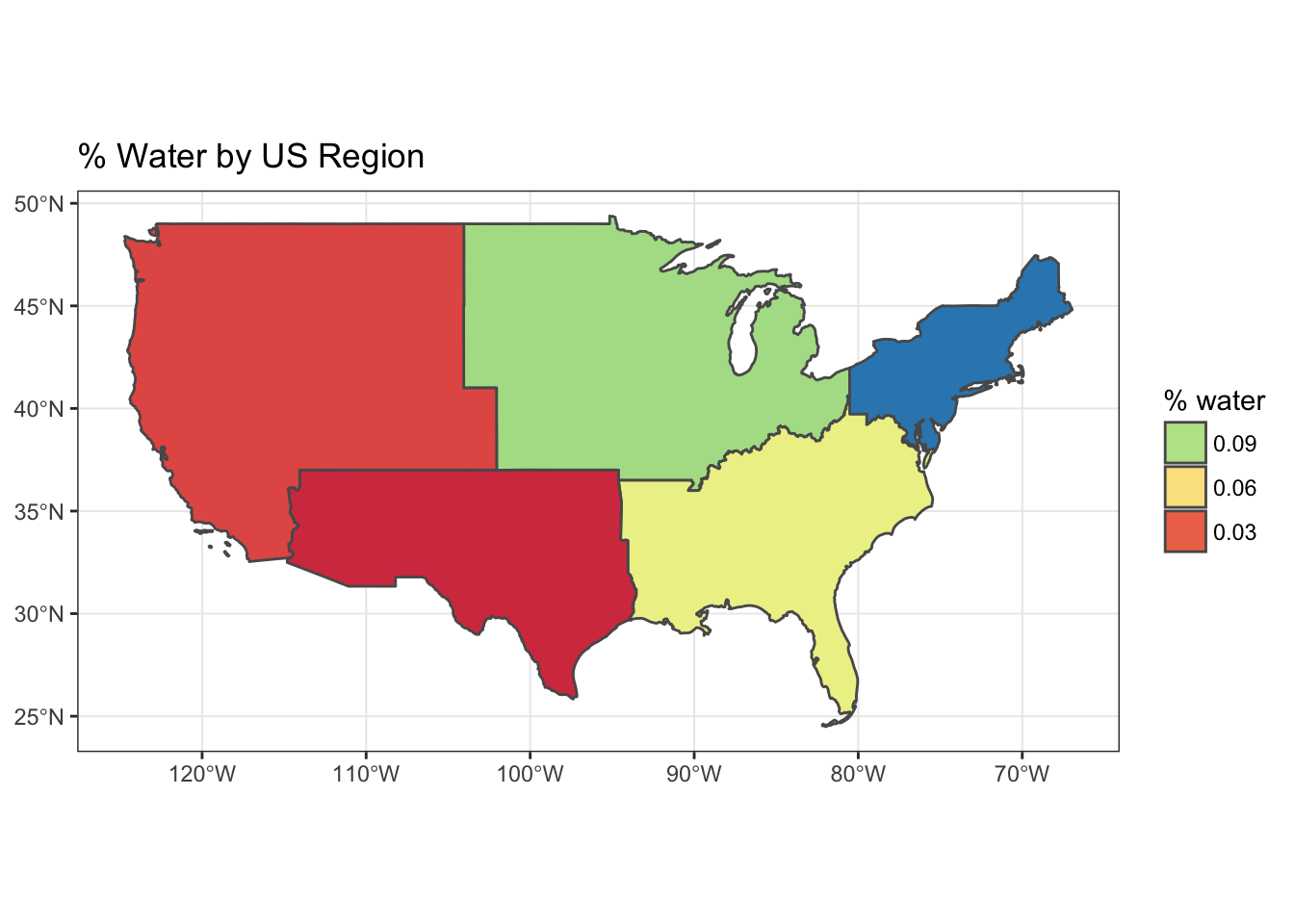## 1.9 Challenge: project & recalculate area

Use `st_transform()` with a USA Contiguous Albers Equal Area Conic Projection that minimizes distoration, and then calculate area using the `st_area()` function.

``````library(geosphere)
library(units)

# Proj4 of http://spatialreference.org/ref/esri/usa-contiguous-albers-equal-area-conic/
crs_usa = '+proj=aea +lat_1=29.5 +lat_2=45.5 +lat_0=37.5 +lon_0=-96 +x_0=0 +y_0=0 +ellps=GRS80 +datum=NAD83 +units=m +no_defs'

regions = states %>%
st_transform(crs_usa) %>%
mutate(
water_m2 = AWATER %>% set_units(m^2),
land_m2  = geometry %>% st_zm() %>% st_area()) %>%
group_by(region) %>%
summarize(
water_m2 = sum(water_m2),
land_m2  = sum(land_m2)) %>%
mutate(
pct_water = water_m2 / land_m2)

# table
regions %>%
st_set_geometry(NULL) %>%
arrange(desc(pct_water))``````
``````## # A tibble: 5 x 4
##      region         water_m2          land_m2    pct_water
##       <chr>          <units>          <units>      <units>
## 1 Northeast 108922434345 m^2 9.117031e+11 m^2 0.11947138 1
## 2   Midwest 184383393833 m^2 1.987266e+12 m^2 0.09278246 1
## 3 Southeast 103876652998 m^2 1.427078e+12 m^2 0.07278973 1
## 4      West  57568049509 m^2 2.467167e+12 m^2 0.02333367 1
## 5 Southwest  24217682268 m^2 1.483758e+12 m^2 0.01632185 1``````
``````# plot, ggplot
ggplot(regions) +
geom_sf(aes(fill = as.numeric(pct_water))) +
scale_fill_distiller("pct_water", palette = "Spectral") +
theme_bw() +
ggtitle("% Water (geodesic) by US Region")``````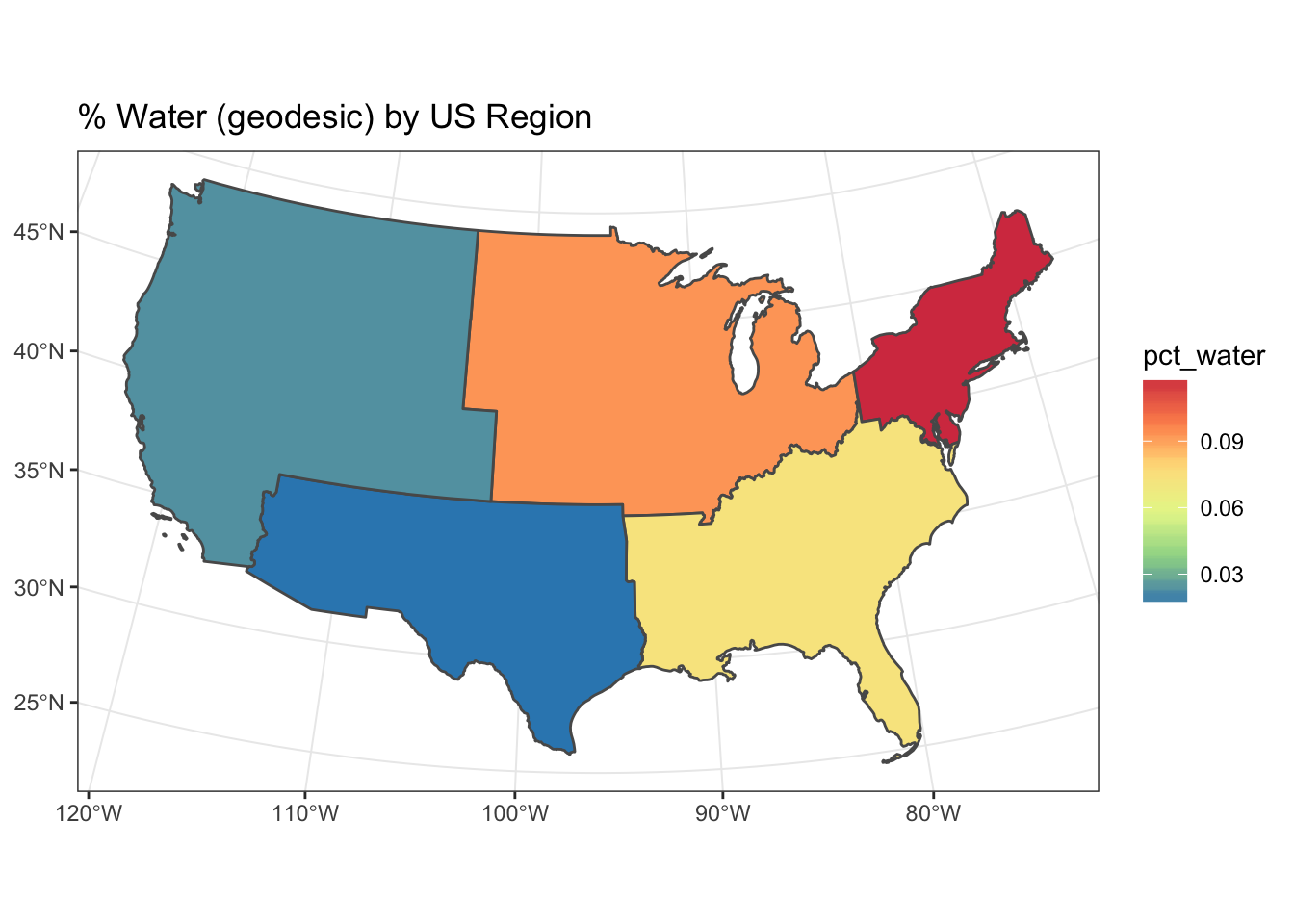## 1.10 Key Points

• The `sf` package can take advantage of chaining spatial operations using the `%>%` operator.
• Data manipulation functions in `dplyr` such as `group_by()`, `summarize()` and `mutate()` work on `sf` objects.
• Area can be calculated a variety of ways. Geodesic is preferred if starting with geographic coordinates (vs projected).